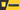### You don't know JavaScript Yet:#9 限制範疇曝光## 最少曝光原則

POLP要求系統中的每一個元件只能訪問當下它所需要的訊息或者資源，以最小的權限、最少的訪問與最少的曝光為原則，達到鞏固整個系統安全，若某個元件故障，對其他元件的影響也會降到最低。

• 命名衝突(Naming Collisions):若在程式中兩個不同地方使用了共享範疇(例如全域範疇)裡面的變數/函式，那麼就會發生命名衝突，如果其中一個地方使用的方式不符合另外一個地方的預期，就容易產生Bug。例如，假設程式中所有的`for`迴圈都共用全域範疇中的變數`i`，很湊巧的我有一個函式A它使用了這個`i`進行迴圈，而迴圈裏頭呼叫了另外一個函式B，而這函式B也使用了全域範疇中的`i`，那麼這個迭代的結果肯定不符合預期。
• 意外行為(Unexpected Behavior):若將某個本該隸屬於私有(private)的變數/函式公開於外部給其他範疇使用，這意味著其他開發人員可能會以你意想不到的方式使用，這將會違反預期上的行為導致Bug。例如，有一個陣列是以`number`型別儲存，但有人將其修改為以`bool`或者`string`的型別，那麼那些意想不到的行為也隨之浮現。
• 無意的依賴(Unintended Dependencys):若你不必要地公開變數/函式，這等同於邀請其他開發人員使用與依賴這些私有的變數/函式，儘管沒有發生上述意外行為的問題，但在將來面對到重構時，你將不能像以前一樣任意的修改變數/函式，這可能會導致你間接了破壞其他開發人員的程式，因為那些並不在你的掌控之中。例如，假設你的程式原本依賴於一個陣列是以`number`型別儲存，但後來你認為它應該使用其他資料結構，但因為其他開發人員也是遵循你當初的設計，這時你就必須負起修改所有受影響程式碼的責任。

``````function diff(x,y) {
if (x > y) {
let tmp = x;
x = y;
y = tmp;
}

return y - x;
}

diff(3,7);      // 4
diff(7,5);      // 2``````

## 隱藏於範疇之中

``````var cache = {};

function factorial(x) {
if (x < 2) return 1;
if (!(x in cache)) {
cache[x] = x * factorial(x - 1);
}
return cache[x];
}

factorial(6);
// 720

cache;
// {
//     "2": 2,
//     "3": 6,
//     "4": 24,
//     "5": 120,
//     "6": 720
// }

factorial(7);
// 5040``````

``````// outer/global scope

function hideTheCache() {
// "middle scope", where we hide `cache`
var cache = {};

return factorial;

// **********************

function factorial(x) {
// inner scope
if (x < 2) return 1;
if (!(x in cache)) {
cache[x] = x * factorial(x - 1);
}
return cache[x];
}
}

var factorial = hideTheCache();

factorial(6);
// 720

factorial(7);
// 5040``````

``````var factorial = (function hideTheCache() {
var cache = {};

function factorial(x) {
if (x < 2) return 1;
if (!(x in cache)) {
cache[x] = x * factorial(x - 1);
}
return cache[x];
}

return factorial;
})();

factorial(6);
// 720

factorial(7);
// 5040``````

### Immediately Invoked Functions Expressions(IIFE)

``````// outer scope

(function(){
// inner hidden scope
})();

// more outer scope``````

### 另類的IIFE

``````!function thisIsAnIIFE(){
// ..
}();

+function soIsThisOne(){
// ..
}();``````

`!``+`和其他幾個運算子都可以用來放在函式前面，將其轉換為函式表達式，在最後使用`()`呼叫就使其為IIFE。

### 函式邊界

IIFE為一個完整的函式，所以在使用時要小心是否產生非預期的結果，例如，若在IIFE中使用`return``this``break``continue`等操作，這些都無法去控制外部範疇中的行為，也就是說如果你有一個函式其中包含了一個IIFE，若這IIFE裡面有使用`return`，只是表示IIFE這個函式的回傳值，而不是外面包覆函式的行為。

## 界定區塊範疇

``````{
// not necessarily a scope (yet)

// ..

// now we know the block needs to be a scope
let thisIsNowAScope = true;

for (let i = 0; i < 5; i++) {
// this is also a scope, activated each
// iteration
if (i % 2 == 0) {
// this is just a block, not a scope
console.log(i);
}
}
}
// 0 2 4``````

• 創立物件(Object)時，可以透過在`{..}`語句中加入key-value作為它的屬性/方法，但這不算是一個範疇。
• 類別(class)使用`{..}`語句將其屬性/方法包覆其中，這也不算是一個範疇。
• `function`宣告中的`{..}`它屬於函式的單一語句，技術上來說它不算是區塊範疇而是函式範疇。
• `switch`語句中的`{..}`不是區塊範疇。

``````if (somethingHappened) {
// this is a block, but not a scope
{
//TDZ of msg start.
// this is both a block and an explicit scope
let msg = somethingHappened.message(); //TDZ of msg end.
notifyOthers(msg);
}
// ..
recoverFromSomething();
}``````

`if`語句中我們為變數`msg`創建了一個區塊範疇，因為整個`if`語句中不需要用到該變數，大多數的開發人員(包括我自己在內)都會很直覺的將變數的控制放在`if`區塊中，若是只有幾行程式碼，可以根據個人的主觀來判斷，但隨著程式逐漸增長，這些變數就有過度曝光的問題。

``````function getNextMonthStart(dateStr) {
var nextMonth, year;

{
let curMonth;
[ , year, curMonth ] = dateStr.match(
/(\d{4})-(\d{2})-\d{2}/
) || [];
nextMonth = (Number(curMonth) % 12) + 1;
}

if (nextMonth == 1) {
year++;
}

return `\${ year }-\${
}-01`;
}
getNextMonthStart("2019-12-25");   // 2020-01-01``````

1. 全域範疇有一個識別字，函式`getNextMonthStart(..)`
2. `getNextMonthStart(..)`函式範疇有三個識別字，`dateStr`(參數)，`nextMonth``year`
3. 內在的區塊範疇`{..}`，裡面有一個識別字`curMonth`

### `var`與`let`

``````function diff(x,y) {
if (x > y) {
var tmp = x;    // `tmp` is function-scoped
x = y;
y = tmp;
}

return y - x;
}``````

``````function getStudents(data) {
var studentRecords = [];

for (let record of data.records) {
let id = `student-\${ record.id }`;
studentRecords.push({
id,
record.name
});
}

return studentRecords;
}``````

``````function commitAction() {
do {
let result = commit();
var done = result && result.code == 1;
} while (!done);
}``````

`result`使用`let`沒什麼問題，因為它只被使用於`do...while`中，`done`是比較值得討論的地方，因為`do...while`看不見自己區塊範疇中使用`let`宣告的變數，所以我們必須使用`var`將其提升(hoisting)至外部範疇使其能看到`done`這個變數。還有另外一種選擇是將`done`宣告於函式範疇中，我想多數人會選擇這種方式，但`done`只用在這個區塊範疇中，透過`var`進行提升的動作或許比較有可讀性。

### 何時使用`let`

``````function diff(x,y) {
var tmp;

if (x > y) {
tmp = x;
x = y;
y = tmp;
}

return y - x;
}``````

``````function diff(x,y) {
if (x > y) {
// `tmp` is still function-scoped, but
// the placement here semantically
// signals block-scoping
var tmp = x;
x = y;
y = tmp;
}

return y - x;
}``````

``````for (var i = 0; i < 5; i++) {
if (checkValue(i)) {
break;
}
}

if (i < 5) {
console.log("The loop stopped early!");
}``````

``````var lastI;

for (let i = 0; i < 5; i++) {
lastI = i;
if (checkValue(i)) {
break;
}
}

if (lastI < 5) {
console.log("The loop stopped early!");
}``````

### 談談`try...catch`

``````try {
doesntExist();
}
catch (err) {
console.log(err);
// ReferenceError: 'doesntExist' is not defined

let onlyHere = true;
var outerVariable = true;
}

console.log(outerVariable);     // true

console.log(err);
// ReferenceError: 'err' is not defined
// ^^^^ this is another thrown (uncaught) exception``````

`err``catch`語句宣告於它的區塊範疇中，若想將訊息與外部溝通，可以透過`var`進行宣告，它將會提升至外部範疇中。

``````try {
doOptionOne();
}
catch {   // catch-declaration omitted
}``````

## Function Declarations in Blocks (FiB)

``````if (false) {
console.log("Does this run?");
}
}

1. `ask()`呼叫失敗，拋出`ReferenceError`，因為它不在外部範疇當中。
2. `ask()`的呼叫導致`TypeError`，代表其識別字`ask`有被提升(hoisting)至外部範疇，但它被定義為`undefined`(因為`if`語句未運行)。
3. `ask()`呼叫成功。

Node也會有這個問題的原因是因為v8引擎先用於瀏覽器中，但Node與瀏覽器共用v8引擎，所以這個異常也存在於Node之中。

``````if (typeof Array.isArray != "undefined") {
function isArray(a) {
return Array.isArray(a);
}
}
else {
function isArray(a) {
return Object.prototype.toString.call(a)
== "[object Array]";
}
}``````

``````if (true) {
console.log("Am I called?");
}
}

if (true) {
}
}

for (let i = 0; i < 5; i++) {
console.log("Or is it one of these?");
}
}

console.log("Wait, maybe, it's this one?");
}``````

``````function isArray(a) {
if (typeof Array.isArray != "undefined") {
return Array.isArray(a);
}
else {
return Object.prototype.toString.call(a)
== "[object Array]";
}
}``````

``````var isArray = function isArray(a) {
return Array.isArray(a);
};

// override the definition, if you must
if (typeof Array.isArray == "undefined") {
isArray = function isArray(a) {
return Object.prototype.toString.call(a)
== "[object Array]";
};
}``````

## 總結

• 盡可能的遵循POLE能防止變數過度的曝光，減少程式的相依性。
• `let``var`使用的選擇根據變數依附於哪個範疇當中(是否使用`var`則視團隊/個人)。
• 避免使用FiB，將函式宣告於區塊之外以避免FiB造成的混淆。